This is an automatically generated documentation by LaTeX2HTML utility. In case of any issue, please, contact us at info@cfdsupport.com.

## System of governing equations in dimensionless form

Lets rewrite the system () into the following form21.6: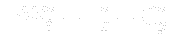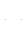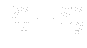(21.23)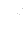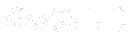(21.24)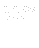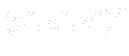(21.25)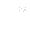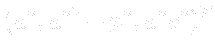(21.26)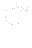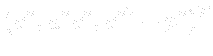(21.27)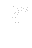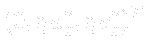(21.28)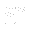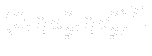(21.29)

where (*) denotes real physical values. Equations () can be transferred to the dimensionless form by following way. Each variable will be divided by its characteristic value of the same dimension.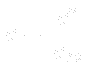(21.30)

Typical characteristic values are: velocity of free-stream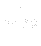and some characteristic distance,e.g. diameter of channel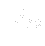. All of the rest variables can be extended with using combination of them: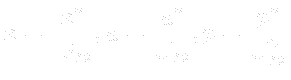(21.31)

After having used this relations and (), the system of governing equations can be written in dimensionless form. Following equations describe flow of incompressible fluid: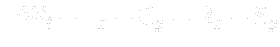(21.32)

where:(21.33)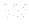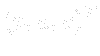(21.34)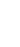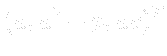(21.35)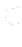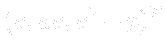(21.36)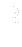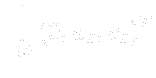(21.37)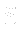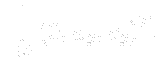(21.38)

where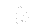is called Reynolds number.

Subsections